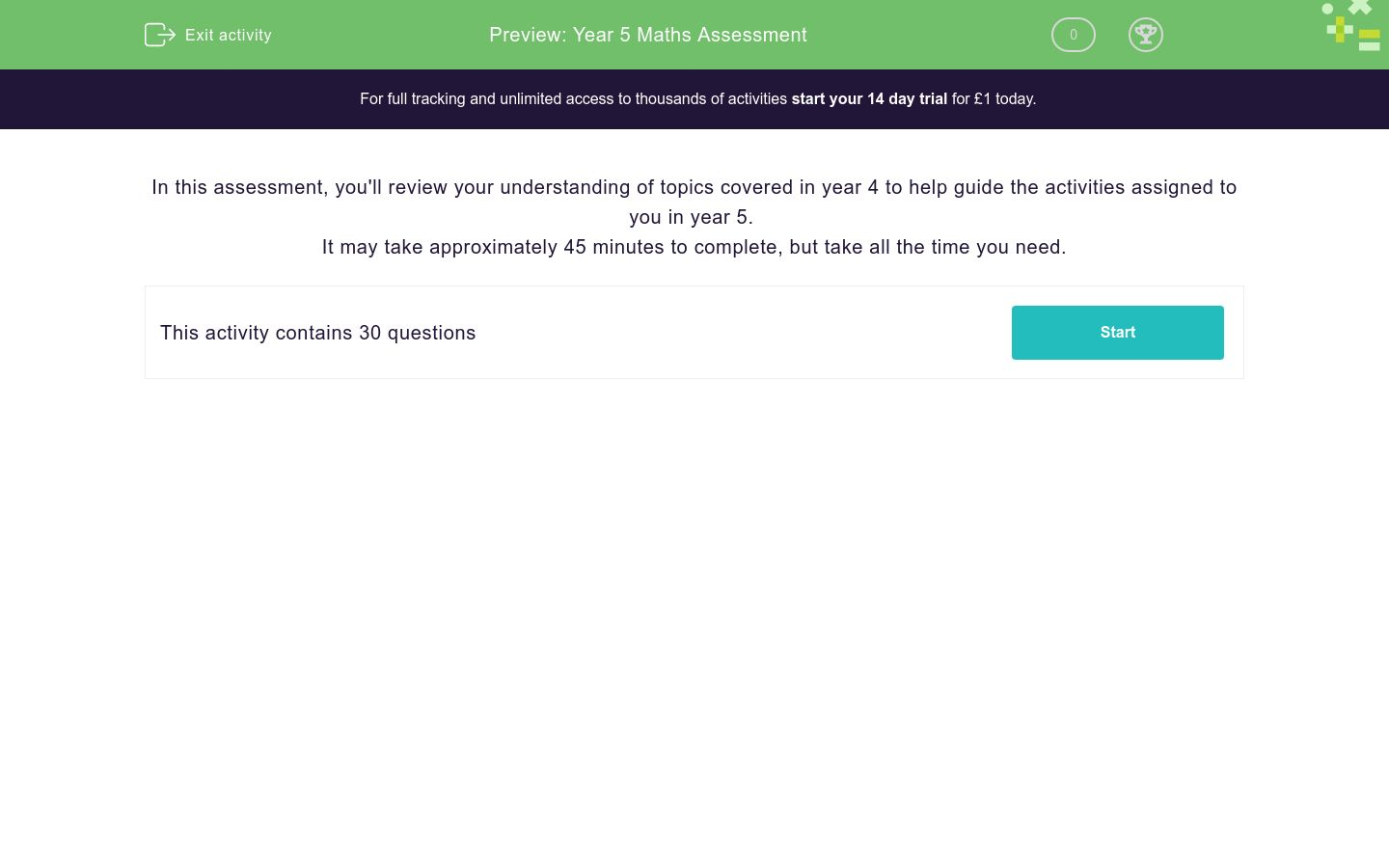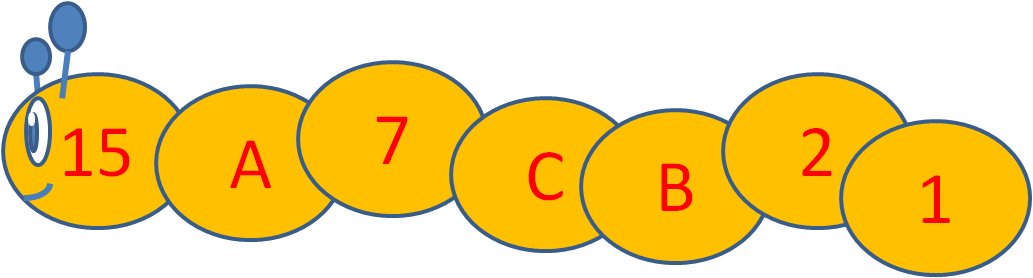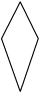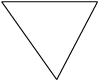# Year 5 Maths Assessment

In this assessment, students will review their comprehension of topics covered at the end of year 4 to help guide their activities and progress in year 5. May take approximately 45 minutes to complete.Key stage:  KS 2

Curriculum topic:   Assessment

Curriculum subtopic:   Starter Assessment

Difficulty level:### QUESTION 1 of 10

In this assessment, you'll review your understanding of topics covered in year 4 to help guide the activities assigned to you in year 5.

It may take approximately 45 minutes to complete, but take all the time you need.

What is the real value of the digit 8 in the number 891 ?

8

80

800

Using four of the digits 8, 6, 9, 1, 9 what are the smallest and largest four-digit numbers that can be made?

 1669 8699 1689 9986 smallest largest

Choose < or > to place between these pairs of numbers.

201 -163
>

<

Match the Roman Numerals to the numbers.

## Column B

92
XLII
42
LXXXVIII
88
XCVIII
98
XCII

Count backwards in 2s to work out the missing number.-22    -24    -26

What is the missing number?

Look at these numbers which go up in 25s.250    275    300

What is the missing number?

If the number is even, divide it by 2.

If the number is odd, subtract 1 from it.

Stop when you get to the number 1.

Work out the values of A, B and C in this number worm, using the rule above.## Column B

A
14
B
6
C
3

 + 1 2 7 3 2 1 4 0

Is the answer to this sum odd or even?

46 + 11 + 47

Odd

Even

Subtract the numbers.

769 - 99 = ?

Jamir says that

5555 - 3587 =
 1968

Use addition to check if he is right or wrong.

right

wrong

We know that 620 - 436 = 184.

Which other facts can we get from this?

436 + 184 = 620

436 - 184 = 620

184 + 436 = 620

184 + 620 = 436

620 - 184 = 436

Find the number that makes the sum correct.

13 + __ = 200

Tick all the factor pairs of:

108

1 and 108

9 and 12

2 and 54

3 and 36

4 and 27

6 and 18

Work out

253 × 5

What is... ?

81 ÷ 9

Use the factor pair 8 × 7 = 56 to work out

560 ÷ 7

Count up in hundredths to work out the missing number.30.5    30.51    30.52

What is the missing number?

 + 4 . 8 6 2 . 5 2 1 . 8 3

Round this decimal number to the nearest whole number:

50.8

Write the missing number.

 3 + 15 = 18 24 24 ?

Use the number line to help you convert this fraction into a decimal amount.

75¾34
×  12  =

Each of Amir's four children get £4.30 pocket money every week.

How much does this cost Amir in pounds every week?

Just write the number.

﻿Calculate the area of this shape, made of centimetre squares and half squares.What is the length of one side of this regular pentagon in cm?Perimeter: 20 cm

Which of these shapes are not right-angled triangles?abcdefgh
a

b

c

d

e

f

g

h

Look at this regular star (pentagram).

Which of the lines are lines of symmetry?a

b

c

d

e

On the same set of axes...

Follow these instructions to plot points and draw lines.

Name the shape that is formed.

Join (8, 0) to (10, 2).

Join (10, 2) to (8, 4).

Join (8, 4) to (6, 2).

Join (6, 2) to (8, 0).

isosceles triangle

right-angled triangle

square

rectangle

parallelogram

Use the table of results to answer the question.

How many more grey cars than red cars passed in total over Monday and Wednesday?• Question 1

What is the real value of the digit 8 in the number 891 ?

800
EDDIE SAYS
The 8 is in the hundreds column.
• Question 2

Using four of the digits 8, 6, 9, 1, 9 what are the smallest and largest four-digit numbers that can be made?

 1669 8699 1689 9986 smallest largest
EDDIE SAYS
The smallest number you can make is 1689 and the largest is 9986. Remember, you can't make 1669 from 8, 6, 9, 1, 9.
• Question 3

Choose < or > to place between these pairs of numbers.

201 -163
>
EDDIE SAYS
Positive numbers are all bigger than negative numbers.
• Question 4

Match the Roman Numerals to the numbers.

## Column B

92
XCII
42
XLII
88
LXXXVIII
98
XCVIII
EDDIE SAYS
92 = 90 + 2 = XC + II = XCII
42 = 40 + 2 = XL + II = XLII
88 = 50 + 38 = L + XXXVIII = LXXXVIII
98 = 90 + 8 = XC + VIII = XCVIII
• Question 5

Count backwards in 2s to work out the missing number.-22    -24    -26

What is the missing number?

-20
EDDIE SAYS
-20... -22... -24... -26
• Question 6

Look at these numbers which go up in 25s.250    275    300

What is the missing number?

225
EDDIE SAYS
225... 250... 275... 300
• Question 7

If the number is even, divide it by 2.

If the number is odd, subtract 1 from it.

Stop when you get to the number 1.

Work out the values of A, B and C in this number worm, using the rule above.## Column B

A
14
B
3
C
6
EDDIE SAYS
15 is odd, so subtract 1 to get A.
7 is odd, so subtract 1 to get C.
Halve C to get B, since C is even.
• Question 8

 + 1 2 7 3 2 1 4 0
3413
EDDIE SAYS
Add up the columns, starting from the ones. Carry if the answer is 10 or more.
• Question 9

Is the answer to this sum odd or even?

46 + 11 + 47

Even
EDDIE SAYS
Add up the ones' digits to get 6 + 1 + 7 = 14, which ends in a 4, which is even.
• Question 10

Subtract the numbers.

769 - 99 = ?

670
EDDIE SAYS
Subtract 100 and then add 1 back.
• Question 11

Jamir says that

5555 - 3587 =
 1968

Use addition to check if he is right or wrong.

right
EDDIE SAYS• Question 12

We know that 620 - 436 = 184.

Which other facts can we get from this?

436 + 184 = 620
184 + 436 = 620
620 - 184 = 436
EDDIE SAYS
Adding is the opposite of subtracting.
Remember that we can add in reverse too.
• Question 13

Find the number that makes the sum correct.

13 + __ = 200

187
EDDIE SAYS
Work out 200 - 13.
• Question 14

Tick all the factor pairs of:

108

1 and 108
9 and 12
2 and 54
3 and 36
4 and 27
6 and 18
EDDIE SAYS
108 = 1 × 108 = 2 × 54 = 3 × 36 = 4 × 27 = 6 × 18 = 9 × 12
• Question 15

Work out

253 × 5

1265
EDDIE SAYS• Question 16

What is... ?

81 ÷ 9

9
EDDIE SAYS
9 × 9 = 81
• Question 17

Use the factor pair 8 × 7 = 56 to work out

560 ÷ 7

80
EDDIE SAYS
8 × 7 = 56
80 × 7 = 560
560 ÷ 7 = 80
• Question 18

Count up in hundredths to work out the missing number.30.5    30.51    30.52

What is the missing number?

30.49
EDDIE SAYS
30.49... 30.5 or 30.50... 30.51... 30.52
• Question 19

 + 4 . 8 6 2 . 5 2 1 . 8 3
9.21
EDDIE SAYS
Add in columns, starting at the right. Carry if the answer is 10 or more. Keep the decimal points lined up.
• Question 20

Round this decimal number to the nearest whole number:

50.8

51
EDDIE SAYS
50.8 is between 50 and 51 but closer to 51.
• Question 21

Write the missing number.

 3 + 15 = 18 24 24 ?
24
EDDIE SAYS
24ths added to 24ths stay as 24ths.
• Question 22

Use the number line to help you convert this fraction into a decimal amount.

75¾75.75
• Question 23
 34
×  12  =
9
EDDIE SAYS
¼ of 12 = 12 ÷ 4 = 3
Multiply this by 3 to get ¾ of 12.
• Question 24

Each of Amir's four children get £4.30 pocket money every week.

How much does this cost Amir in pounds every week?

Just write the number.

17.20
17.2
EDDIE SAYS
£4.30 × 4 = £17.20
• Question 25

﻿Calculate the area of this shape, made of centimetre squares and half squares.9
EDDIE SAYS
There are 2 × 4 = 8 whole centimetre squares.
There are also 2 half-squares.
8 + 1 = 9
• Question 26

What is the length of one side of this regular pentagon in cm?Perimeter: 20 cm
4cm
4 cm
4
EDDIE SAYS
20 ÷ 5 = 4
• Question 27

Which of these shapes are not right-angled triangles?abcdefgh
a
b
c
d
e
g
EDDIE SAYS
f and h are triangles with right angles inside.
• Question 28

Look at this regular star (pentagram).

Which of the lines are lines of symmetry?a
b
c
d
e
EDDIE SAYS
A regular pentagram has 5 lines of symmetry from each point to the opposite 'indent'.
• Question 29

On the same set of axes...

Follow these instructions to plot points and draw lines.

Name the shape that is formed.

Join (8, 0) to (10, 2).

Join (10, 2) to (8, 4).

Join (8, 4) to (6, 2).

Join (6, 2) to (8, 0).

square
EDDIE SAYSStill a square even though it has been turned.
• Question 30

Use the table of results to answer the question.

How many more grey cars than red cars passed in total over Monday and Wednesday?20
EDDIE SAYS
(24 + 33) - (21 + 16) = 57 - 37 = 20
---- OR ----

Sign up for a £1 trial so you can track and measure your child's progress on this activity.

### What is EdPlace?

We're your National Curriculum aligned online education content provider helping each child succeed in English, maths and science from year 1 to GCSE. With an EdPlace account you’ll be able to track and measure progress, helping each child achieve their best. We build confidence and attainment by personalising each child’s learning at a level that suits them.

Get started